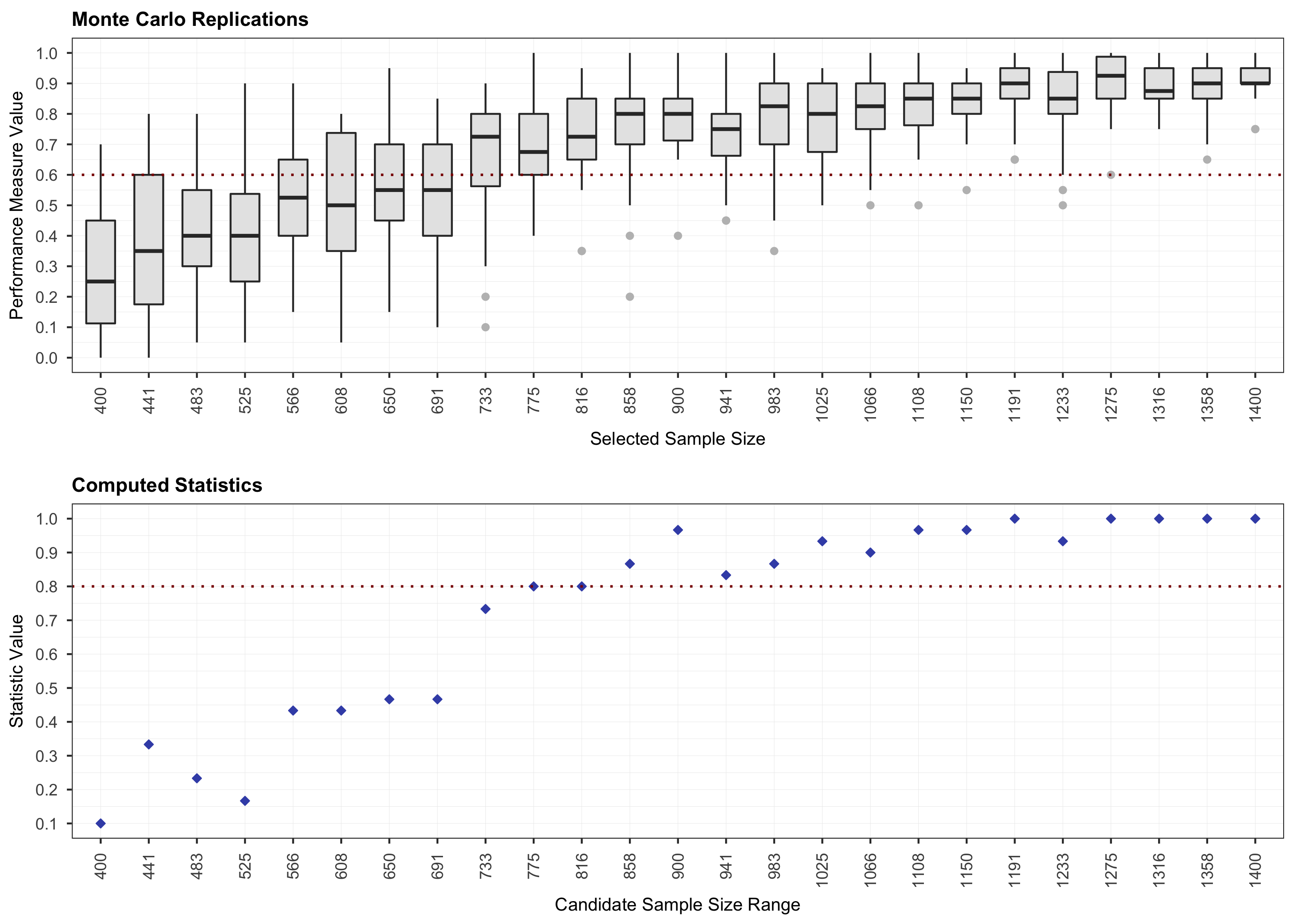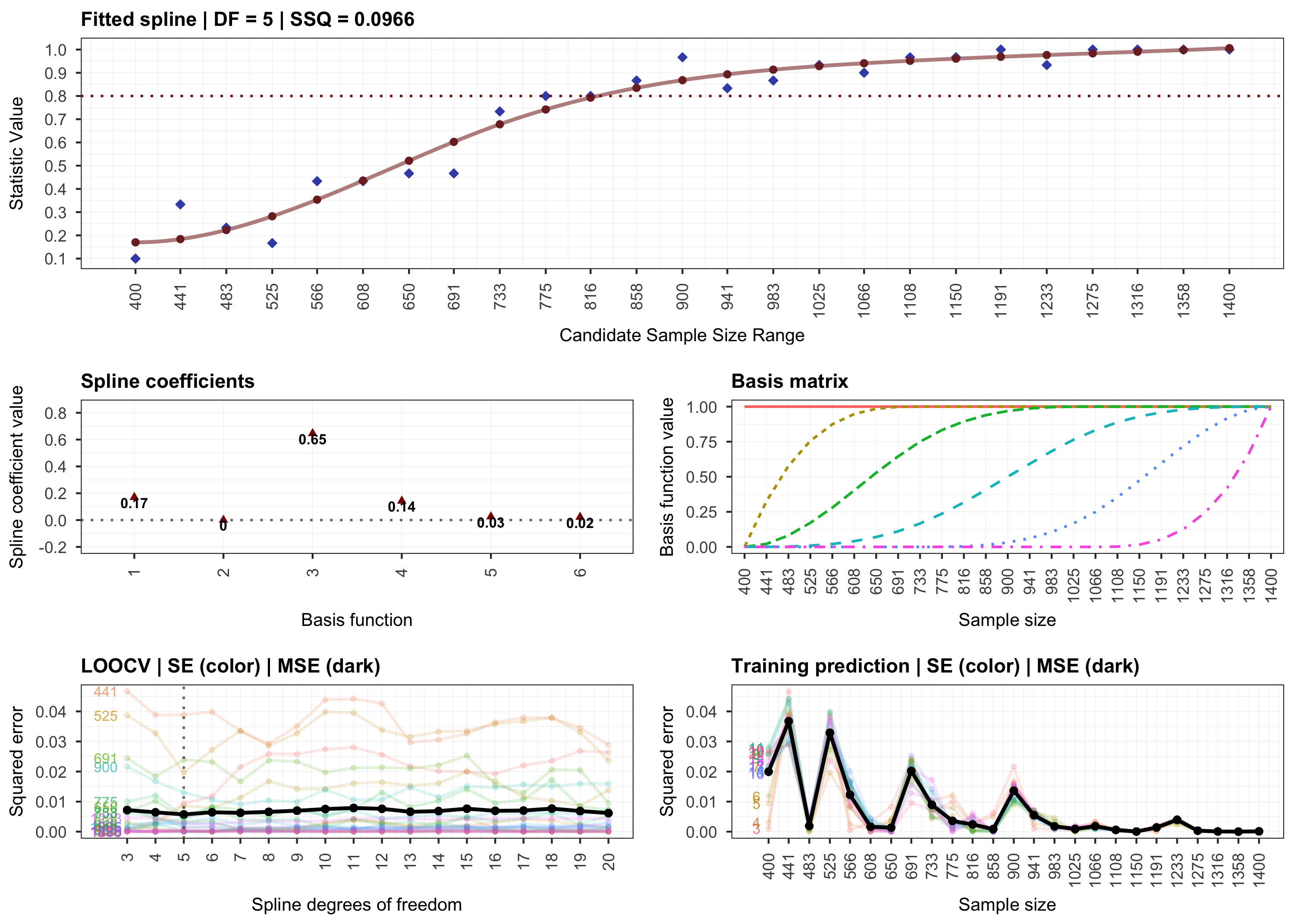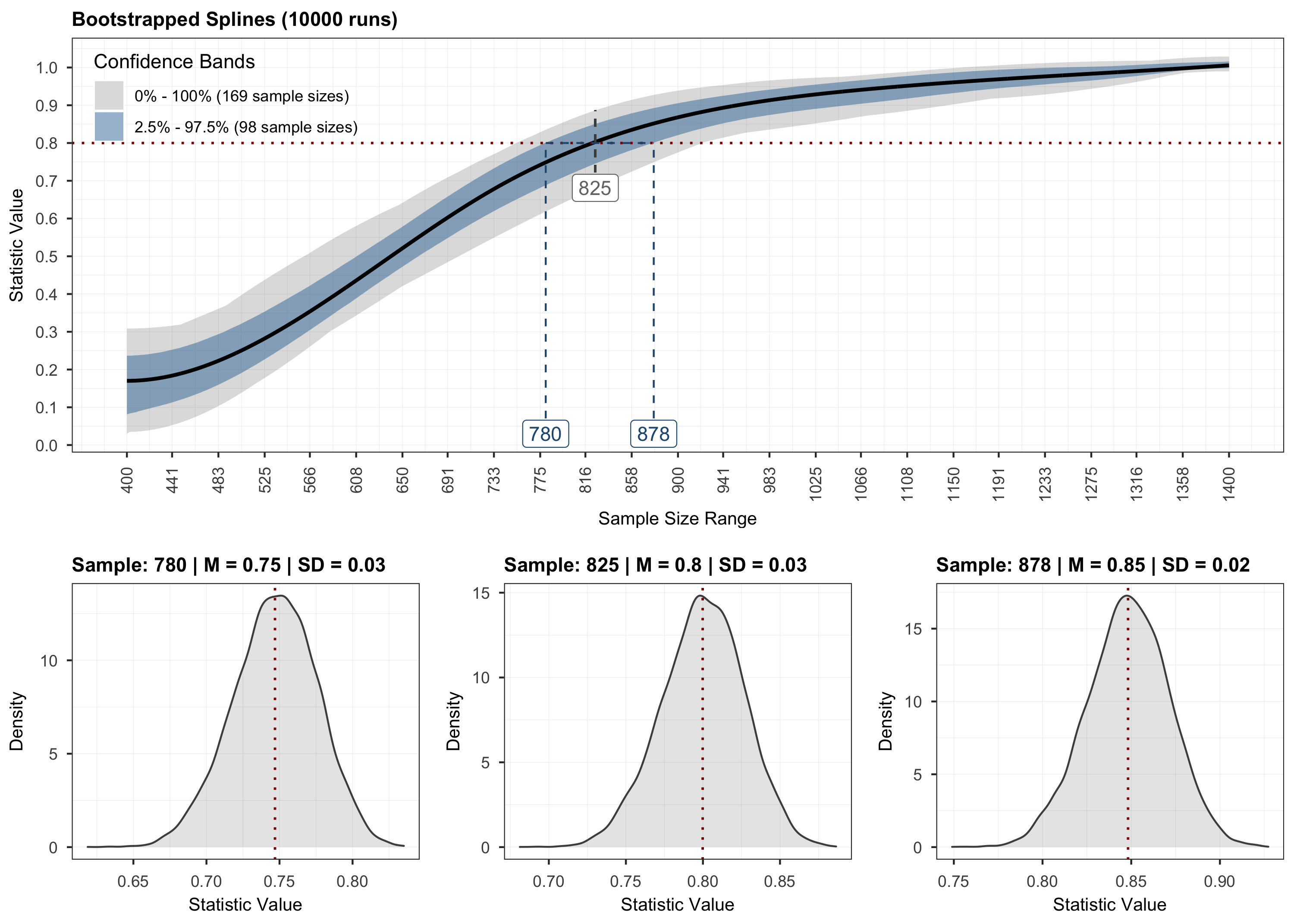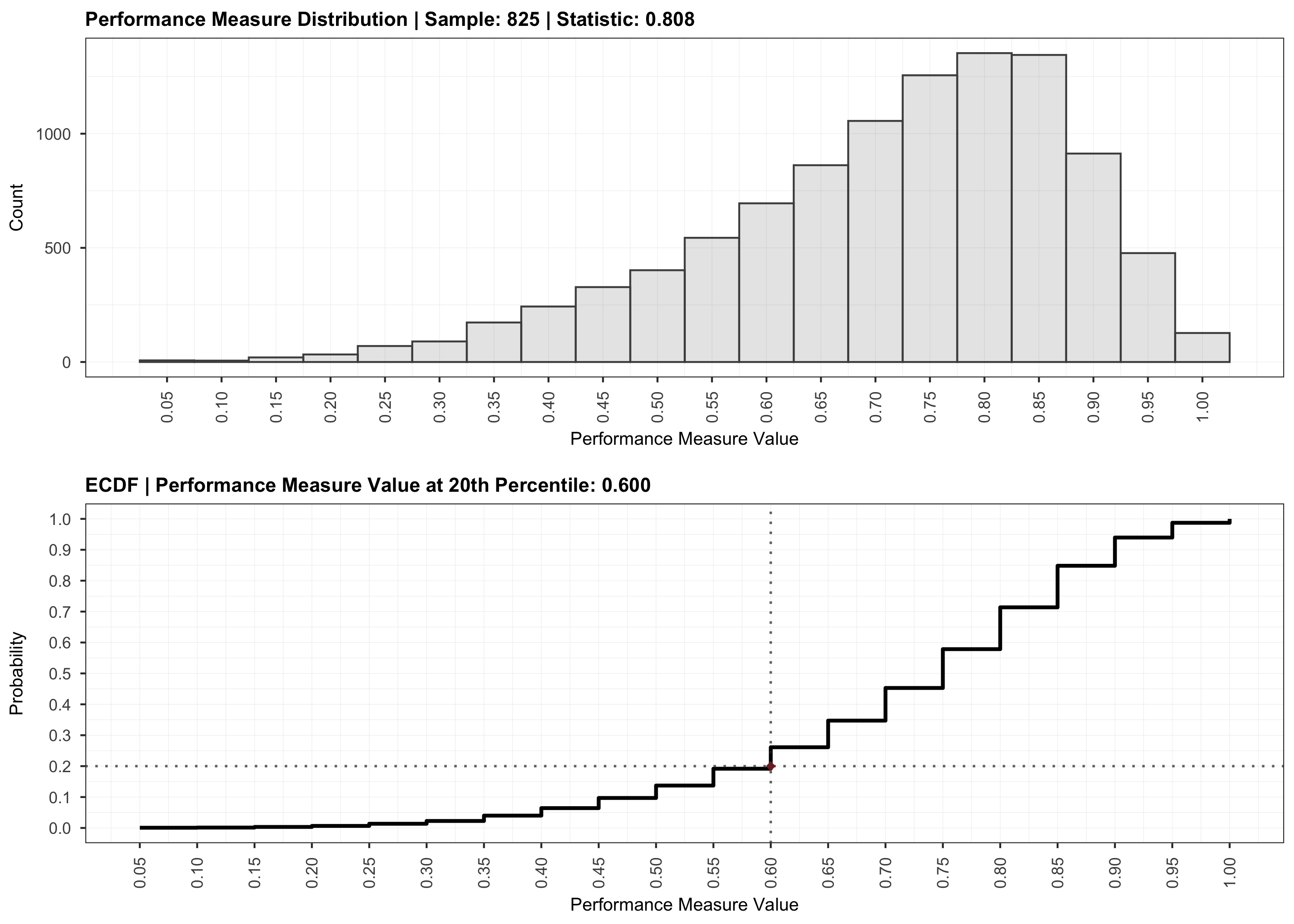# Sample Size Analysis for Psychological Networks …and more

## Description

`powerly` is an `R` package that implements the method by Constantin et al. (2021) for conducting sample size analysis for cross-sectional network models. The method implemented is implemented in the main function `powerly`()`. The implementation takes the form of a three-step recursive algorithm designed to find an optimal sample size value given a model specification and an outcome measure of interest. It starts with a Monte Carlo simulation step for computing the outcome at various sample sizes. Then, it continues with a monotone curve-fitting step for interpolating the outcome. The final step employs stratified bootstrapping to quantify the uncertainty around the fitted curve.

``````Check out the documentation and tutorials at
<h3>
<a href="https://powerly.dev">powerly.dev</a>
</h3>``````

## Installation

• to install from CRAN run `install.packages("powerly")`
• to install the latest version from GitHub run `remotes::install_github("mihaiconstantin/powerly")`

## Example

The code block below illustrates the main function in the package. For more information, see the documentation `?powerly`, or check out the tutorials at powerly.dev.

``````# Suppose we want to find the sample size for observing a sensitivity of `0.6`
# with a probability of `0.8`, for a GGM true model consisting of `10` nodes
# with a density of `0.4`.

# We can run the method for an arbitrarily generated true model that matches
# those characteristics (i.e., number of nodes and density).
results <- powerly(
range_lower = 300,
range_upper = 1000,
samples = 30,
replications = 20,
measure = "sen",
statistic = "power",
measure_value = .6,
statistic_value = .8,
model = "ggm",
nodes = 10,
density = .4,
cores = 2,
verbose = TRUE
)

# Or we omit the `nodes` and `density` arguments and specify directly the edge
# weights matrix via the `model_matrix` argument.

# To get a matrix of edge weights we can use the `generate_model()` function.
true_model <- generate_model(type = "ggm", nodes = 10, density = .4)

# Then, supply the true model to the algorithm directly.
results <- powerly(
range_lower = 300,
range_upper = 1000,
samples = 30,
replications = 20,
measure = "sen",
statistic = "power",
measure_value = .6,
statistic_value = .8,
model = "ggm",
model_matrix = true_model, # Note the change.
cores = 2,
verbose = TRUE
)``````

To validate the results of the analysis, we can use the `validate()` method. For more information, see the documentation `?validate`.

``````# Validate the recommendation obtained during the analysis.
validation <- validate(results)``````

To visualize the results, we can use the `plot` function and indicate the step that should be plotted.

``````# Step 1.
plot(results, step = 1)````````````# Step 2.
plot(results, step = 2)````````````# Step 3.
plot(results, step = 3)````````````# Validation.
plot(validation)``````## Contributing

• To support a new model, performance measure, or statistic, please open a pull request on GitHub.
• To request a new model, performance measure, or statistic, please open an issue on GitHub. If possible, also include references discussing the topics you are requesting.

## PosterTo use `powerly` please cite: - Constantin, M. A., Schuurman, N. K., & Vermunt, J. (2021). A General Monte Carlo Method for Sample Size Analysis in the Context of Network Models. PsyArXiv. https://doi.org/10.31234/osf.io/j5v7u